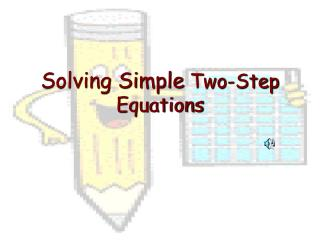# Solving Simple Two-Step Equations - PowerPoint PPT PresentationDownload PresentationSolving Simple Two-Step Equations

Solving Simple Two-Step EquationsDownload Presentation## Solving Simple Two-Step Equations

- - - - - - - - - - - - - - - - - - - - - - - - - - - E N D - - - - - - - - - - - - - - - - - - - - - - - - - - -
##### Presentation Transcript

1. Solving Simple Two-Step Equations

2. What is a Two-Step Equation? An equation that requires 2 operations on each side to isolate the variable. REMEMBER: THE GOAL IS TO ISOLATE THE VARIABLE

3. Examples of Two-Step Equations • 3x – 5 = 16 • y/4 + 3 = 12 • 5n + 4 = 6 • n/2 – 6 = 4

4. Steps for Solving Two-Step Equations • Solve for any Addition or Subtraction on the variable side of equation by “undoing” the operation from both sides of the equation. • Solve any Multiplication or Division from variable side of equation by “undoing” the operation from both sides of the equation.

5. Addition  Subtraction Opposite Operations Multiplication  Division

6. Helpful Hints? • Identify what operations are on the variable side. (Add, Sub, Mult, Div) • “Undo” the operation by using opposite operations. • Whatever you do to one side, you must do to the other side to keep equation balanced.

7. Ex. 1: Solve 4x – 5 = 11 4x – 5 = 15 +5 +5 (Add 5 to both sides) 4x = 20 (Simplify) 4 4 (Divide both sides by 4) x = 5 (Simplify)

8. Try These Examples • 2x – 5 = 17 • 3y + 7 = 25 • 5n – 2 = 38 • 12b + 4 = 28

9. Check your answers!!! • x = 11 • y = 6 • n = 8 • b = 2